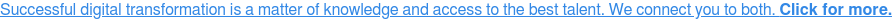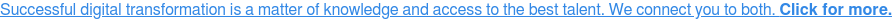# Funny things happened on the way to the forum

Data scientists are all experts in statistics, right? Wrong. In Statistical Mistakes Even Scientists Make I review some common statistical errors committed by scientists. Yes, scientists do make statistical mistakes. Lots of ‘em.

What about data science, then? The definition of a data scientist is highly imprecise and includes IT professionals, programmers, AI researchers, statisticians and folks we used to just call scientists. By most definitions I know, I am a data scientist.To keep things tidy, however, in this brief article I’ll narrow my definition of data scientist to non-statisticians working in data mining and predictive analytics. This is not a small group.

Many working in this area have had little or no formal education in statistics and are heavily focused on data wrangling and simple predictive modelling. These predictive models may be well-established statistical methods such as OLS linear regression and binary logistic regression, or more recent “machine learners” such as XGBoost.

What follows are my impressions based on social media blogs and posts, articles in the business media, YouTube podcasts, textbooks and papers on data science, and numerous interactions with other data scientists.

Regrettably, fundamental statistical errors, misunderstandings, and substandard practice do not appear infrequent, and many other statisticians I know who work in data mining and predictive analytics have offered similar assessments.1. Many data scientists seem to associate Bayesian statistics with naïve Bayes and Bayesian networks. However, any statistical model, including familiar ones such as linear and logistic regression, can also be conducted within a Bayesian framework.
2. Principal components analysis is widely used in data science and predictive analytics, but I seldom see components rotated. Rotation can greatly enhance interpretation of the components and help us spot irrelevant or redundant variables.
3. In K-means clustering there are many ways to choose initial seeds, but random selection appears to be the only way many data scientists know. Likewise, K-means is not restricted to Euclidean distance, and there are at least two dozen other distance/similarity measures we can choose from. Again, many data scientists do not seem aware of this.
4. Dummy coding (“one-hot coding”) seems to be the main method known for representing categorical data. Effect coding, for example, is rarely used in data science. This article from the UCLA Institute for Digital Research and Education is a concise summary of eight ways to code categorical data.
5. In situations where we need to analyze multiple dependent variables simultaneously, multiple models are typically run when one would have been more efficient and better statistically. Structural Equation Modeling, for instance, can accommodate multiple dependent variables of mixed data types, either as observed variables or indicators of a latent construct.
6. Understanding of experimental designs seems limited. For example, I rarely see factorial designs employed in data science. In addition, there are numerous quasi-experimental designs popular in fields such as epidemiology and psychology that are seldom used. There are many excellent textbooks on these subjects, such as Experimental Design: Procedures for the Behavioral Sciences (Kirk) and Experimental and Quasi-Experimental Designs (Shadish et al.)
7. Knowledge of inferential statistics tends to be thin. As a result, much time and effort can be wasted working with gigantic data files when small samples would suffice. Moreover, when sampling is used, it is almost always simple random or systematic (every nth) sampling, and stratified, cluster and other sampling schemes seem infrequent.
8. Logistic regression models are typically considered classifiers, though classification was not their original purpose. When logistic regression is used for classification, group sizes should be considered, and the standard software default of 0.50 may not be appropriate. Moreover, there can be multiple cutoffs. For instance, it might make business sense to split predicted probability of loan default into three groups, e.g., Low (under 0.20), Moderate (0.20-.79), and High (0.80 or more).
9. Data are often treated as either binary or numeric, though statisticians have developed numerous models for count, ordinal and multinomial data.
10. Use of multiple models where one would be enough appears common. For instance, say we have a dependent variable comprised of five types of consumers. We do not need to run five binary models (each of the five types versus all others), let alone ten models (all possible pairs). A single omnibus model, such as multinomial logistic or probit regression, would be all we’d need in most cases.
11. The term multilevel is often used to mean a model with an outcome variable which has more than two categories. In statistics, multilevel means something altogether different and that form of model is rarely made use of in data mining and predictive analytics (though it would often be sensible). Polytomous variables can be nominal or ordinal and there are many statistical procedures for these sorts of outcomes.
12. Main-effects regression models are very popular, but it is far less common to see terms to account for interactions and curvilinear relationships included on the right-hand side of the equation. There seems to be little awareness of quantile regression, regression splines, generalized additive models and other regression methods widely used in statistics as alternatives to linear regression.
13. While there is growing interest in times-series analysis, knowledge of it seems mostly confined to simple univariate forecasting. There are an enormous number of time-series models besides these elementary types.
14. When I was in graduate school, data mining was synonymous with data dredging. Personally, I don’t feel it’s wise to boast about torturing data until it confesses. Data are notorious for recanting their confessions at inopportune times!
15. There seems to be little interest in interpreting data. Should we be “passionate about data” or have a strong professional interest in what data mean to decision makers? Likewise, little attention is paid to analysis of causation in data science.

To be fair, statistics is more complex and challenging than many may believe. I’ve been studying and using it for more than 30 years and learn something new about it every day. Statistics is hard to teach and getting harder because so many new methods are being developed while, at the same time, older ones are being extended or modified.Also, courses on statistics are frequently taught by non-statisticians, and one consequence is that bad habits are passed from one generation to the next.

Fortunately, statistics is now a hot field and academic statisticians have taken a strong interest in data mining and predictive analytics. It will take time, but bad habits can be broken, and ways of thinking changed.

Browse

Article by channel:

Read more articles tagged: Analytics, Featured